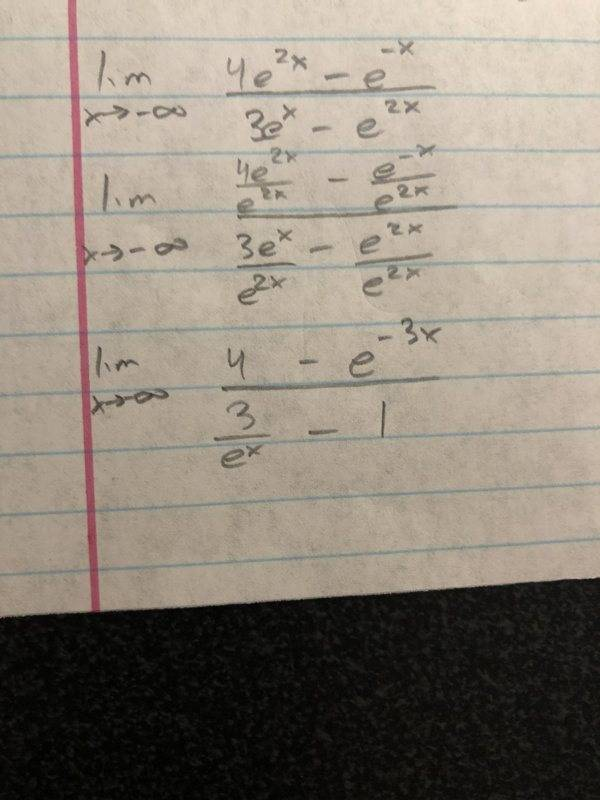# Can someone help me with this limit where x approaches - infinity?

newguy_13
Homework Statement:
Limit problem
Relevant Equations:
There are none
I don't know what do do from here other than i can make the 3/e^x a 0 due to the fact its divided by such a large number. What do i do with the e^-3x? Thanks for the helpLast edited by a moderator:

Mentor
2022 Award
Homework Statement: Limit problem
Homework Equations: There are none

I don't know what do do from here other than i can make the 3/e^x a 0 due to the fact its divided by such a large number. What do i do with the e^-3x? Thanks for the help
The same. What happens to ##e^{-3x}## if ##x## gets larger and larger? What does ##e^{-sth.}## mean?

newguy_13
So the answer is just -4?

Homework Helper
Gold Member
2022 Award
So the answer is just -4?
You're looking for the limit as ##x## tends to ##-\infty##.

Mentor
2022 Award
You must be careful if you cancel factors, as they can tend to zero and then you lose information which one is faster. It is always better to avoid divisions as long as possible. That means: Simplify the initial quotient by considering ##x \to -\infty##, then you get one single term ##e^{...x}## which tells you the result.

newguy_13
Ok so can you let me know what the answer is? That would help me to understand where i went wrong and what to do in the future problems. Thanks!

Homework Helper
Gold Member
2022 Award
Ok so can you let me know what the answer is? That would help me to understand where i went wrong and what to do in the future problems. Thanks!

You took the limit ##x \rightarrow +\infty##.

Homework Helper
Gold Member
2022 Award
Ok so can you let me know what the answer is? That would help me to understand where i went wrong and what to do in the future problems. Thanks!

Re helping you with the problem. The first thing I would do is look at each of the four terms and see what happens in the limit. The numerator should be clear, but you may need to take a closer look at the denominator.

Mentor
You're looking for the limit as ##x## tends to ##-\infty##.
I think that's what the OP has, but may have misread what he/she wrote in the 3rd line, where the limit part looks a bit like x -->-- .

Homework Helper
Gold Member
2022 Award
I think that's what the OP has, but may have misread what he/she wrote in the 3rd line, where the limit part looks a bit like x -->-- .

Yes, but the OP has calculated the terms based on ##x \rightarrow +\infty##.

So the answer is just -4?

Mentor
2022 Award
Ok so can you let me know what the answer is?
Not finite. Look at the recipe in post #6, only two steps.

Homework Helper
We have

$lim_{x\rightarrow -\infty}\frac{4e^{2x}-e^{-x}}{3e^{x}-e^{2x}}=lim_{x\rightarrow -\infty}\frac{4e^{2x}-\frac{1}{e^{x}}}{e^{x}\left( 3-e^{x}\right) }=lim_{x\rightarrow -\infty}\frac{4e^{3x}-1}{e^{2x}\left( 3-e^{x}\right) }$

then $\left( 4e^{3x}-1\right) \rightarrow -1$ and $\left( 3-e^{x}\right)\rightarrow 3$ as $x\rightarrow -\infty$ but $\frac{1}{e^{2x}}\rightarrow$ ? as $x\rightarrow -\infty$?

•Delta2
Gold Member
We have

$lim_{x\rightarrow -\infty}\frac{4e^{2x}-e^{-x}}{3e^{x}-e^{2x}}=lim_{x\rightarrow -\infty}\frac{4e^{2x}-\frac{1}{e^{x}}}{e^{x}\left( 3-e^{x}\right) }=lim_{x\rightarrow -\infty}\frac{4e^{3x}-1}{e^{2x}\left( 3-e^{x}\right) }$

then $\left( 4e^{3x}-1\right) \rightarrow -1$ and $\left( 3-e^{x}\right)\rightarrow 3$ as $x\rightarrow -\infty$ but $\frac{1}{e^{2x}}\rightarrow$ ? as $x\rightarrow -\infty$?
##\frac {1}{e^{2x}}## will go to +##\ infty## as ## x \rightarrow \infty##. Denominator will become indefinitely small.

Homework Helper
@WWGD I know this, I was attempting to walk the OP thru it. Sorry to highjack your thread, I just like to feel useful somehow. Cheers!

•Delta2